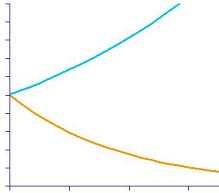If a sum invested gains 10% each year how long will it be before it has doubled its value?

If an object depreciates in value by 10% each year how long will it take until only half of the original value remains?

Why aren’t these two answers the same?

Is there a rate, used for both gain and depreciation, for which those two answers would actually be the same?

See the notes for teachers and solution and also http://nrich.maths.org/5636

Tagged with: